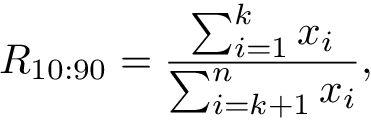Coefficients to measure inequality.

# Inequality Coefficients:

This is small library with some implemented coefficients (or indices) intended to measure inequality or concentration of the values in a population.

## Implemented coefficients

• Gini Coefficient:

• Ordinary. Follows this formula:• Corrected. Uses a correction for small datasets based on Deltas, 2003.
• Ratio top / rest. Follows this formula:Where k is is the ceil value for 100 - percentage you define. For instance, if you take k = 10, you are getting the ratio of inequality between the top 10% percentage and the rest 90% percentage. In particular, this specific value of k is given to you directly by the ratio_top10_rest() function.

## Installation

This library is hosted on PyPI, so installation is straightforward. The easiest way to install type this at the command line (Linux, Mac, or Windows):

pip install inequality_coefficients


This library also depends on numpy, but pip should take of that for you already.

## Basic Usage

For the simplest, typical use cases, this tells you everything you need to know.:

import inequality_coefficients as ineq
data = array([1.7, 3.2 ...]) # data can be list of nums or numpy array
gini_coeff = ineq.gini(data)
ratio_top_rest = ineq.ratio_top10_rest(data)


# Development

To setup the development environment install all the dev dependiencies with pip install -r requirements.txt and install the latest version in your sites-packages with python setup.py develop.

## Run tests

I use pytest. Install it with pip install -U pytest and run the test with the development setup with pytest.

## Acknowledgements

Firstly, I was based on Felipe Ortega's wikixray code for implementing the gini coefficient, however, my code has changed so much (I have even fixed a bug in his code) and also now I'm using numpy as backend.

Anyway, I want to thank him for open sourcing that project.

## Project details

This version1.2.31.2.21.2.11.2.01.1.11.1.01.0.11.0.0

Uploaded source